# Yang-Yang anomaly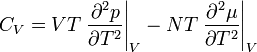$C_V = VT \left. \frac{\partial^2 p}{\partial T^2} \right\vert_V -NT \left. \frac{\partial^2 \mu}{\partial T^2} \right\vert_V$
where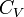$C_V$ is the heat capacity at constant volume and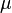$\mu$ is the chemical potential. Given that experimentally it is found that$C_V$ diverges at the critical temperature this implies that either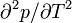$\partial^2 p/\partial T^2$ or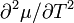$\partial^2 \mu/\partial T^2$, or both, diverge as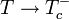$T \rightarrow T_c^-$. Fisher and Orkoulas  showed that both terms diverge.